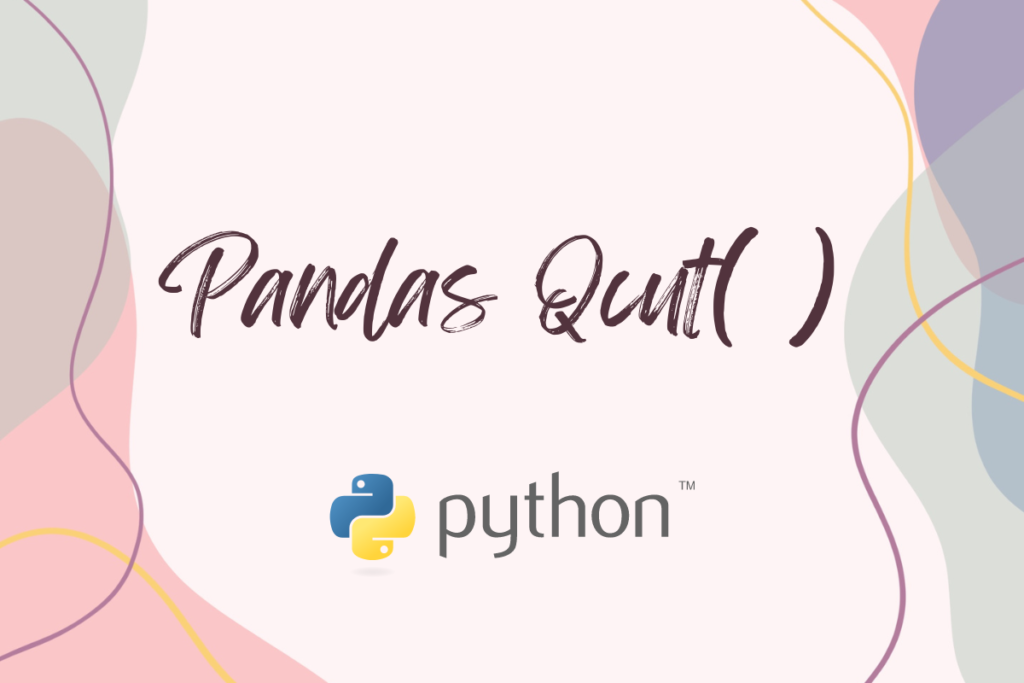# Pandas qcut – An Easy Explanation with ExamplesThere is already an article in AskPython covering the aspects of the pandas cut() function. If you haven’t read it yet, please try it out. Believe me, it would be a worthy read 😉.

So, when the pandas library already has a function to cut why bother with another by the name, pandas qcut( )?

This is exactly what this article sets out to explore. Further, it details the differences between both the cut functions within the pandas library and demonstrates the capability of the qcut( ) function with a handful of examples.

So, let us get started by importing the pandas library using the below code.

```import pandas as pd
```

Thereafter, the nuances of the pandas qcut( ) function shall be explored through each of the below sections.

• Pandas qcut( ) Vs Pandas cut( )
• Syntax of the qcut( ) function
• Use cases for the qcut( ) function

## Pandas qcut( ) Vs Pandas cut( )

While both functions help in segmenting a given dataset and sorting them into different bins, there is a subtle difference that sets them apart. The total count of entities in each bin would more or less be the same when a dataset is put through the qcut( ) function. This results in a difference in the size of the intervals at which the data is segmented.

On the other hand, when pandas cut( ) is used to obtain bin sizes of equal intervals but with a different count of entities in each bin. So, there you go! The difference between the different cut functions within the pandas library is for your eyes only.

Now you know that, let us get on with understanding deeper, the qcut( ) function.

## Syntax of the qcut( ) function

The qcut( ) is a quantile-based discretization function which discretizes a given dataset into bins of equal size based on rank or sample quantiles. Following is its syntax detailing the mandatory and optional constituents required for its functioning.

```pandas.qcut( x, q, Labels=None, retbins=False, precision=3, duplicates=’raise’)
```

where,

• One-dimensional array or a series that is to be cut.
• q Number of quantiles into which the input dataset is to be cut.
• Labels – Set to ‘None’ by default, it is used to specify the names for the resulting bins.
• retbins – Set to ‘False’ by default, it is used to specify whether or not to return bins.
• precision – Set to ‘3’ by default, it is used to specify the number of decimal digits that is to be returned.
• duplicates – Set to ‘raise’ by default, it is used to specify how to deal with the duplicity in bin edges. It raises an error when set to ‘raise’ & removes the duplicate bin edge when set to ‘drop’.

## Use cases for the qcut( ) function

The following dataframe shall be used to demonstrate the functioning of qcut( ).

```df = pd.DataFrame({'score':[60, 87, 49, 51, 69, 74, 92, 55, 63, 78, 47, 86]})
```

Now let us try to cut the data across the quartiles.

```Catg = pd.qcut(df['score'], 4)
Print(Catg)
```
```Output:
0       (54.0, 66.0]
1       (80.0, 92.0]
2     (46.999, 54.0]
3     (46.999, 54.0]
4       (66.0, 80.0]
5       (66.0, 80.0]
6       (80.0, 92.0]
7       (54.0, 66.0]
8       (54.0, 66.0]
9       (66.0, 80.0]
10    (46.999, 54.0]
11      (80.0, 92.0]
Name: score, dtype: category
Categories (4, interval[float64, right]): [(46.999, 54.0] < (54.0, 66.0] < (66.0, 80.0] < (80.0, 92.0]]
```

It is evident from the above result that the count of entities within each bin is the same. Let us now use the same dataset & try to specify the quantiles along with putting a cap on the number of decimal places.

```Catg = pd.qcut(df['score'], q=[0, 0.4, 0.8, 1], precision = 0)
print (Catg)
```

One can also add labels to the above result, should the need arise.

```Catg = pd.qcut(df['score'], q=[0, 0.4, 0.8, 1], labels=["Poor", "Average", "Excellent"])
print (Catg)
```